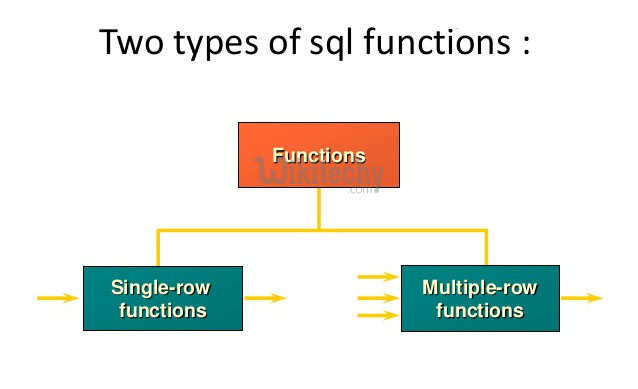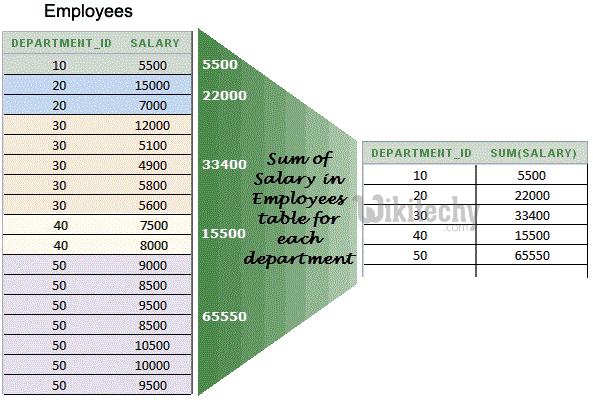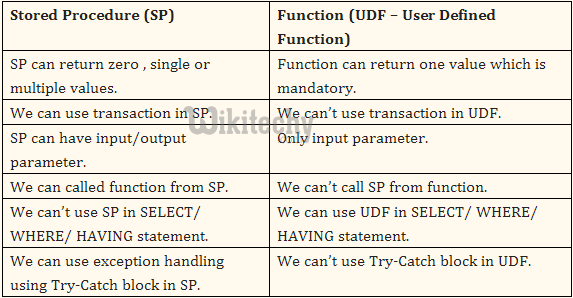# Oracle Functions - oracle tutorial - sql tutorial

## What is Oracle Functions ?

• SQL functions are built into Oracle and are available for use in various appropriate SQL statements.
• You can also create your own function using PL/SQL.## Single-Row Functions

• Single-row functions return a single result row for every row of a queried table or view. These functions can appear in select lists, WHERE clauses, START WITH and CONNECT BY clauses, and HAVING clauses.
• The Oracle SQL Functions are divided into five types, they are
• Number Functions (Math Functions)
• Character Functions
• Miscellaneous Functions
• Aggregate Functions
• Date and Time Functions

## Number Functions:

• Number functions accept numeric input and return numeric values.
• Most of these functions return values that are accurate to 38 decimal digits.

## Character Functions.

• A character function is a function that takes one or more-character values as parameters and returns either a character value or a number value.
• The Oracle Server and PL/SQL provide a number of different character datatypes.
• Including CHAR, VARCHAR, VARCHAR2, LONG, RAW, and LONG RAW.

## Miscellaneous Functions:

• The Miscellaneous Functions available in Oracle SQL functions are,

## COALESCE

• Coalesce function returns the first not null value in the expression list.

## DECODE

• Decode functions compares an expr with search value one by one.
• If the expr does not match any of the search value then returns the default value. If the default value is omitted then returns null.

## GREATEST:

• Returns the greatest expr from an expr list.

## LEAST:

• It is simillar to greatest. It returns the least expr from the expression list.

## NVL:

• This function is oftenly used to check null values.
• It returns expr2 if the expr1 is null, otherwise returns expr1.
• NVL2
• NVL2 returns expr2 if expr1 is not null, otherwise return expr3.
• NULLIF
• Nullif compares expr1 with expr2. If they are equal then returns null, otherwise return expr1.

## UID:

• Returns the current session ID of user logged on.
• Returns the username of the current user logged on

## SYS_CONTEXT:

• SYS_CONTEXT returns the value of parameter associated with the context namespace.
• You can use this function in both SQL and PL/SQL statements.

## VSIZE:

• Returns the internal representation of expr in bytes.

## Multi-Row Functions:

• Multi-Row Functions (also called group or aggregate functions) return a single value based on groups of rows, rather than single value for each row.
• You can use Aggregate functions in select lists and in ORDER BY and HAVING clauses.
• They are commonly used with the GROUP BY clause in a SELECT statement, where Oracle divides the rows of a queried table or view into groups.
• The important Aggregate functions are
• Avg
• Sum
• Max
• Min
• Count
• Stddev
• VarianceOracle sql group by aggregate functions

## avg()

• The avg() function returns the average value of a numeric field from a group of rows. For example,
• returns the average salary of all employees.

## count()

• The count() function counts the number of rows in a group of rows. This function counts all rows in the group, including those for which a NULLvalue is present. There are two ways of calling count(), as follows:
• The first example returns the total number of rows that match the query’s WHERE clause. The second example returns the total number of rows that have a non-NULL value in the specified column.

## max()

• The max() function returns the highest value of a specified column from a group of rows. For example,
• returns the salary of the highest paid employee.

## min()

• The min() function returns the lowest value of a specified column from a group of rows. For example,
• returns the salary of the lowest paid employee.

## sum()

• The sum() function returns the total of all values for a specified column in a group of rows. For example,
• returns the total number of vacation days taken by employees this year.

## Date and Time Functions:

• To see the system date and time use the following functions:

## CURRENT_DATE:

• It returns the current date in the session time zone, in a value in the Gregorian calendar of datatype DATE

## SYSDATE:

• SYSDATE returns the current date and time.

## SYSTIMESTAMP:

• SYSTIMESTAMP function returns the system date, including fractional seconds and time zone of the database.
• The return type is TIMESTAMP WITH TIME ZONE

## Stored Procedure Vs Functions